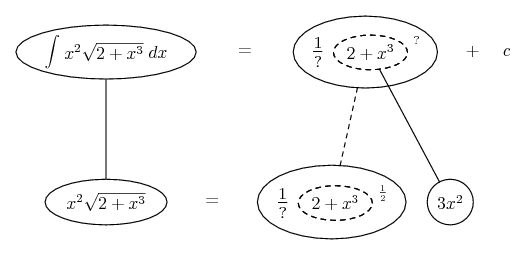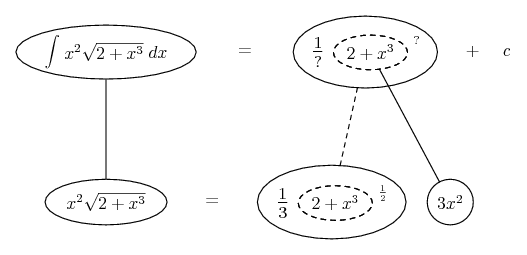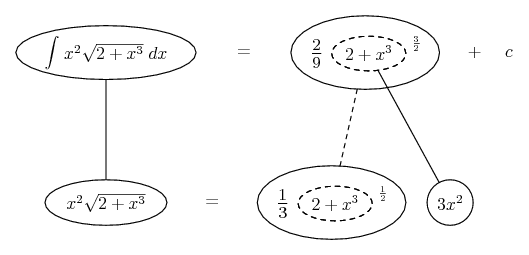# Thread: Indefinate Integral Help

1. ## Indefinate Integral Help

Evaluate: $\displaystyle \int x^2 \sqrt{2 + x^3} dx$

I can't figure out how to do these and my book provides no help. If anyone can give me an example I would appreciate it.

2. Is...

$\displaystyle \frac{d}{dx} (2 + x^{3}) = 3\cdot x^{2}$ (1 )

... so that...

Kind regards

$\displaystyle \chi$ $\displaystyle \sigma$

3. ## SO the Answer

is 3x^2?

4. use the substitusion method,

let $\displaystyle u=2 + x^{3}$
then
$\displaystyle \frac{du}{dx}=3x^{2}$

and solve it . . .

cheers5. And, just in case a picture helps...... where... is the chain rule. Straight continuous lines differentiate downwards (integrate up) with respect to x, and the straight dashed line similarly but with respect to the dashed balloon expression (the inner function of the composite which is subject to the chain rule).

Spoiler:Spoiler:__________________________________________

Don't integrate - balloontegrate!

Balloon Calculus; standard integrals, derivatives and methods

Balloon Calculus Drawing with LaTeX and Asymptote!

#### Search Tags

indefinate, integral